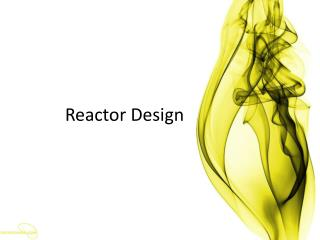DownloadDownload PresentationReactor Design

# Reactor Design

Télécharger la présentation## Reactor Design

- - - - - - - - - - - - - - - - - - - - - - - - - - - E N D - - - - - - - - - - - - - - - - - - - - - - - - - - -
##### Presentation Transcript

1. Reactor Design

2. الجمهور: هو احنا هناخد إيه النهاردة؟ أنا: هناخد Reactor الجمهور: بس؟ أنا: آه بس الجمهور: هو السكشن طويل أنا: آه الجمهور: يعني هناخد السكشن ونص أنا: آه الجمهور: بس احنا تعبانين أنا: وأنا تعبانة أكتر منكم الجمهور: بس احنا صايمين أنا: ربنا يتقبل إن شاء الله الجمهور: طب احنا هناخد إيه في ال Reactor؟ أنا: Combined Reactors الجمهور: بس احنا ما أخدناهاش في المحاضرة؟ وال gas phase هناخده امتى؟ أنا: ماهي بتاخد في السكشن بس ، بعد العيد إن شاء الله

3. Combined Reactors

4. Exercise • Calculate the volume of a PFR and a CSTR required for 90% conversion of reactant by a first-order reaction: . • Calculate the total volume of two CSTRs (both of the same volume) in series required for 90% conversion.

5. Series combination of cstr and pfr

6. When PFRs and CSTRs are both available and to be used in series, the order of the reactors can affect the total conversion, as the conversion will be dependent on the inlet concentration to each reactor. Therefore, the overall will depend on the order in which the reactors are placed. • For first order reactions, the overall conversion will be independent of reactors order.

7. For n > 1, PFR should be placed before CSTR, Why? High rates (at high concentrations) are required in order to maximize the conversion. As PFR takes the advantage of the high reaction rates, it should be placed before CSTR. The reverse occurs when n <1.

8. If we have more than one CSTR of different sizes together with the PFR, the smallest one should be placed immediately after the PFR so as to keep higher concentrations and hence higher rates of reaction.

9. hippopotamus’s digestive system

10. The hippopotamus digestive system is modeled as a CSTR and a PFR in a series. For the system above A represents the grass that makes up the bulk of the hippos normal diet. F is the molar flow of A, m is the mass flow and X represents the conversion of A into proteins, vitamins, minerals and everything else that the hippo needs to survive.

11. Finding correct values of X is of course impossible but fairly good estimates can be done. We further assumed that about 75% of the total conversion occurred in the first part of the digestive system, i.e. the CSTR or the stomach, and 25% in the second part, i.e. the PFR or the intestines. The assumption is based on the volume ratio between the two parts. Studies made of the stomach contents of the hippo are not extensive enough to make any final conclusions but at least the protein contents in different parts of the stomach are in concord with the aforementioned assumption.

12. Sheet 5

13. Pure A is fed at a volumetric flow rate of 5 lit/sec and a concentration of 10 gmole/lit to an existing CSTR which is connected to an existing tubular reactor in series. If the volume of the CSTR is 20 lit, and the tubular reactor volume is 8 lit, what are the intermediate and final conversions that can be achieved with the existing system if the reaction: is first order with k = 1 sec-1. What if the previous system is reversed?

14. Consider the liquid phase reaction: With the rate constant k = 2 sec-1 in a PFR of volume 1 m3. The volumetric flow rate of feed is 0.5 m3/sec of pure A. It is desired to replace the PFR with a cascade of 3 equal volumes CSTR in series while maintaining the same outlet conversion. What volume for each of the three CSTRs would be needed?

15. It is desired to carry out the following first order reaction: (K = 0.1 min-1 at the operating temperature). A CSTR of volume 100 lit is fed at a flow rate of 50 lit/min of fresh feed containing A in the concentration of 5 gmole/lit. The outlet stream from this CSTR is fed to a tubular reactor of total length 100 cm and cross-sectional area of 200 cm2. 25 lit/min of the effluent stream leaving the PFR is recycled again to the CSTR, such that the net product stream from the PFR flows at the rate of 50 lit/min. What are the outlet conversions of the CSTR and the PFR?

16. The kinetics of the aqueous – phase decomposition of A is investigated in two mixed reactors in series, the second having twice the volume of the first reactor. At steady state with a feed concentration of 1 mole A/lit. and mean residence time of 96 sec in the first reactor, the concentration in the first reactor is 0.5 mole A/lit. and in the second is 0.25 mole A/lit. Find the kinetic equation for the decomposition.